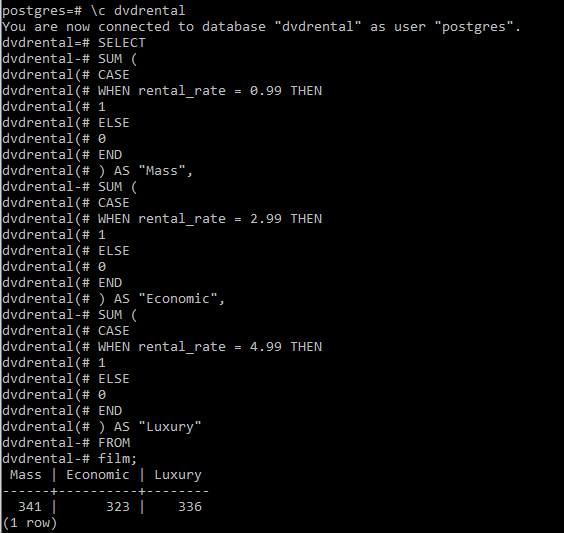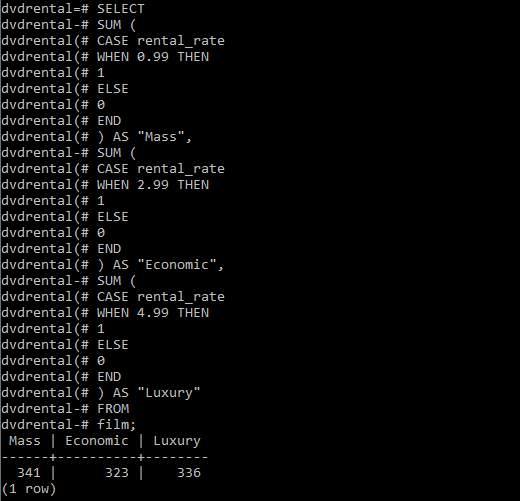# PostgreSQL – CASE

PostgreSQL has a conditional expression called CASE to form conditional queries. The PostgreSQL CASE expression is the same as IF/ELSE statement in other programming languages. PostgreSQL provides two forms of the CASE expressions.

```Syntax:
CASE
WHEN condition_1  THEN result_1
WHEN condition_2  THEN result_2
[WHEN ...]
[ELSE result_n]
END```

For examples we will be using the sample database (ie, dvdrental).

Example 1:
Here we will work on the film table of the sample database. Suppose you want to assign a price segment to a film with the following logic:

• Mass if the rental rate is 0.99
• Economic if the rental rate is 1.99
• Luxury if the rental rate is 4.99

We will query for number of films in each segment using the below statement:

```SELECT
SUM (
CASE
WHEN rental_rate = 0.99 THEN
1
ELSE
0
END
) AS "Mass",
SUM (
CASE
WHEN rental_rate = 2.99 THEN
1
ELSE
0
END
) AS "Economic",
SUM (
CASE
WHEN rental_rate = 4.99 THEN
1
ELSE
0
END
) AS "Luxury"
FROM
film;```

Output:Example 2:
PostgreSQL provides another form of the CASE expression called simple form as follows:

```CASE expression
WHEN value_1 THEN
result_1
WHEN value_2 THEN
result_2
[WHEN ...]
ELSE
result_n
END;```

We can rewrite the general CASE expression using the simple CASE as follows:

```SELECT
SUM (
CASE rental_rate
WHEN 0.99 THEN
1
ELSE
0
END
) AS "Mass",
SUM (
CASE rental_rate
WHEN 2.99 THEN
1
ELSE
0
END
) AS "Economic",
SUM (
CASE rental_rate
WHEN 4.99 THEN
1
ELSE
0
END
) AS "Luxury"
FROM
film;```

Output:
The query returns the same result as the first CASE example.Whether you're preparing for your first job interview or aiming to upskill in this ever-evolving tech landscape, GeeksforGeeks Courses are your key to success. We provide top-quality content at affordable prices, all geared towards accelerating your growth in a time-bound manner. Join the millions we've already empowered, and we're here to do the same for you. Don't miss out - check it out now!

Previous
Next